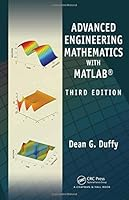Advanced Engineering Mathematics with MATLAB, 3rd Edition2 Reviews
2010-10-26
1105 pages

Book Description

Taking a practical approach to the subject, Advanced Engineering Mathematics with MATLAB®, Third Edition continues to integrate into the conventional topics of engineering mathematics. The author employs MATLAB to reinforce concepts and solve problems that require heavy computation. MATLAB scripts are available for download at www.crcpress.com Along with new examples, problems, and , this updated and expanded edition incorporates several significant improvements.

New to the Third Edition

• New chapter on Green’s
• New section that uses the matrix exponential to solve of differential equations
• More numerical methods for solving differential equations, including Adams–Bashforth and finite element methods
• New chapter on probability that presents basic concepts, such as mean, variance, and probability functions
• New chapter on random processes that focuses on noise and other random fluctuations

Suitable for a differential equations course or a variety of engineering mathematics courses, the text covers fundamental techniques and concepts as well as Laplace transforms, separation of variable solutions to partial differential equations, the z-transform, the Hilbert transform, vector calculus, and . It also highlights many modern applications in engineering to show how these topics are used in practice. A solutions manual is available for qualifying instructors.

Book Details

• Title: Advanced Engineering Mathematics with MATLAB, 3rd Edition
• Author:
• Length: 1105 pages
• Edition: 3
• Language: English
• Publisher:
• Publication Date: 2010-10-26
• ISBN-10: 1439816247
• ISBN-13: 9781439816240